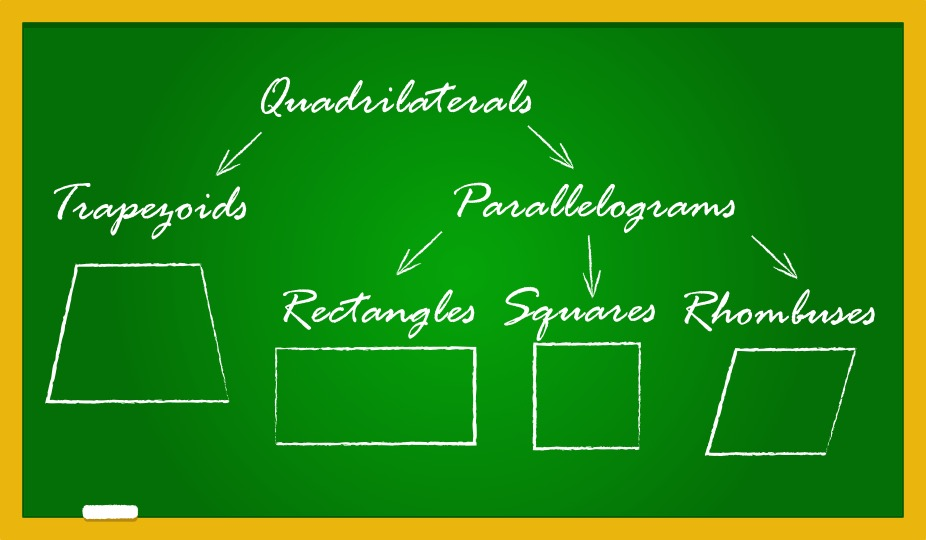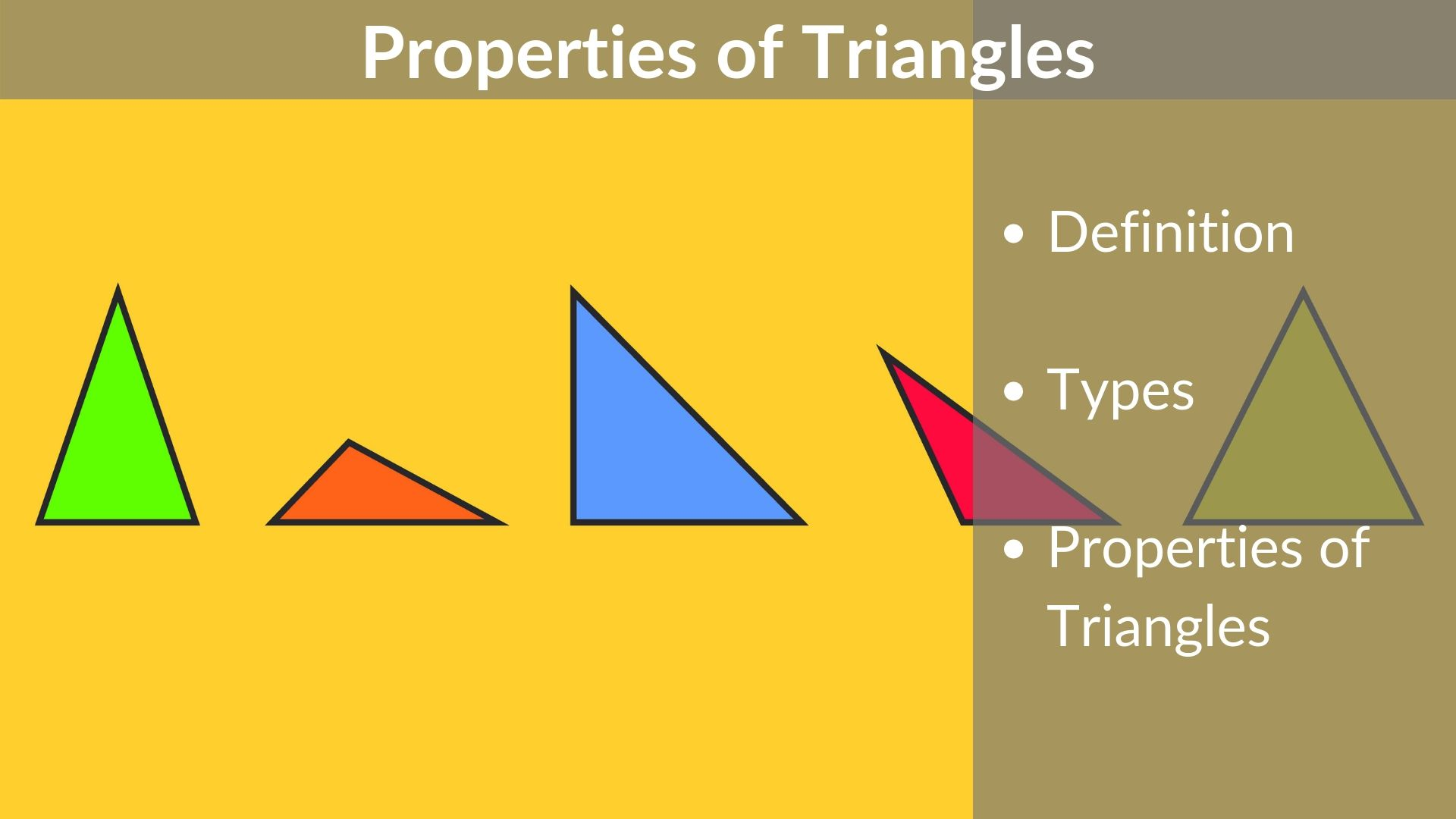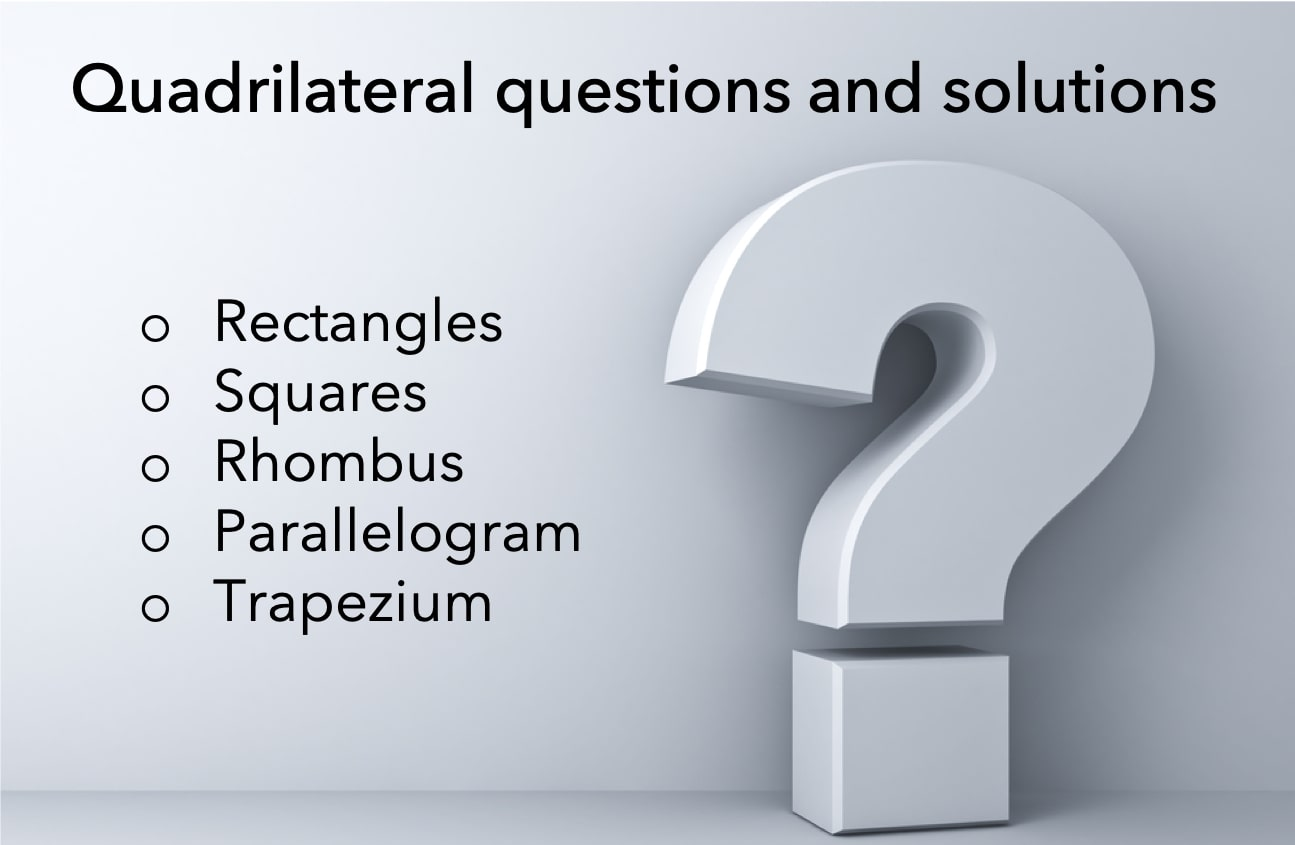# A six-sided mosaic contains 24 triangular pieces of tile of the same size and shape, as shown in the figure above. If the sections of tile fit together perfectly, how many square centimetres of tile are in the mosaic? – OG 2020 Question #329 with Solution

OG 2020: Question No. 329 A six-sided mosaic contains 24 triangular pieces of tile of the same size and shape, as shown in the figure above. If the sections of tile fit together perfectly, how many square centimetres of tile are in the mosaic? Each side of each triangular piece of tile is 9 centimetres

Read More# Lines and Angles – Definitions, Properties, Types, Practice Questions

Ancient mathematicians introduced the concept of lines to represent straight objects which had negligible width and depth. Considered as a breadth less length by Euclid, lines form the basis of Euclidean geometry. When two rays (part of a straight line) intersect each other in the same plane, they form an angle. The point of intersection

Read More# Properties of Quadrilaterals – Rectangle, Square, Parallelogram, Rhombus, Trapezium

In Euclidean geometry, a quadrilateral is a four-sided 2D figure whose sum of internal angles is 360°. The word quadrilateral is derived from two Latin words ‘quadri’ and ‘latus’ meaning four and side respectively. Therefore, identifying the properties of quadrilaterals is important when trying to distinguish them from other polygons. So, what are the properties

Read More# Properties of Circle | Circle Formulas – Area and Perimeter

A circle is a closed shape formed by tracing a point that moves in a plane such that its distance from a given point is constant. The word circle is derived from the Greek word kirkos, meaning hoop or ring. in this article, we cover the important terms related to circles, their properties, and various

Read More# Properties of triangle – Important formulas and classification

In this article, we are going to learn about the simplest form of a polygon, a triangle. All polygons can be divided into triangles, or in other words, they are formed by combining two or more triangles. Thus, understanding the basic properties of triangle and their types is essential. Here is an outline of the

Read MoreQuadrilateral questions have always been a head-scratcher for a lot of candidates taking competitive or standardized exams. The primary reason behind it is learning a concept is easier as compared to applying that concept to solve questions. Therefore, to help you with the application of properties of quadrilaterals we have shared a few quadrilateral questions

Read More# On a scale drawing, a rectangle 1 inch by 1(1/3) inches represents the floor of a room and the indicated scale is 1-inch equals 15 feet. How many square tiles 6 inches on a side will be needed to cover this floor? (1 foot = 12 inches) – OG 2020 Question #100 with Solution

[two_third] OG 2020: Question No. 100 On a scale drawing, a rectangle 1 inch by 1(1/3) inches represents the floor of a room and the indicated scale is 1-inch equals 15 feet. How many square tiles 6 inches on a side will be needed to cover this floor? (1 foot = 12 inches) 40 70

Read More# In the floor plan of an executive’s beach house above, the north and south walls of the living room are parallel. What is the floor area, in square feet, of the bedroom? – OG 2020 Question #99 with Solution

[two_third] OG 2020: Question No. 99 In the floor plan of an executive’s beach house above, the north and south walls of the living room are parallel. What is the floor area, in square feet, of the bedroom? 450√3 450 225√3 225 It cannot be determined from the information given. [/two_third] [one_third_last] Source OG 2020

Read More# Three-fourths of the area of a rectangular lawn 30 feet wide by 40 feet long is to be enclosed by a rectangular fence. If the enclosure has full width and reduced length rather than full length and reduced width, how much less fence will be needed? – OG 2020 Question #98 with Solution

Read MoreRead More